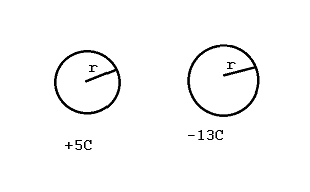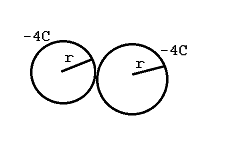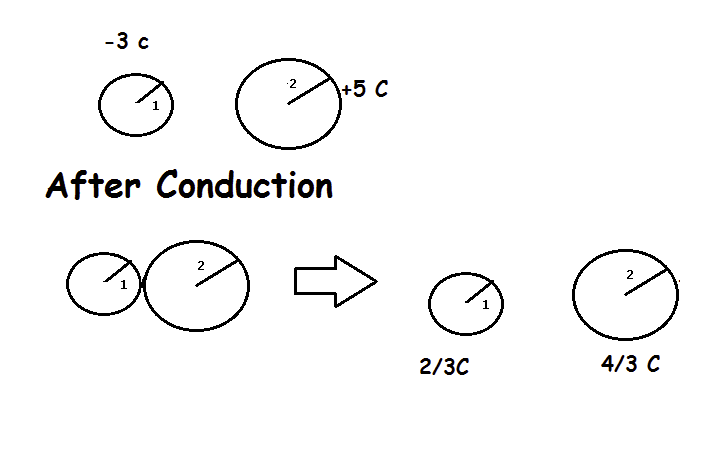# Conduction Short Trick Physics for JEE & NEET – 7tricks

Here is the awesome trick to solve physics questions related to Conduction in just seconds!
Just read this carefully:-

★Case 1:

If the conductors having same radius then :-
Do algebraic sum of charges on both conductors and divide it by two and you will get the answer.

After conduction charge on both conductors will be =
(charge on 1st + charge on 2nd)/2

Let’s learn with some examples:
Charge on first conductor= +5C
Charge on 2nd conductor = -13C
So after conduction Final charge on both
5 + (-13) /2  = -4 C
So the Final answer is -4 C

★Case 2:
If radius of both the conductors are different.
Let you have two conductors having radius R1 and R2 respectively .
Then you need to use this formula to get the final charge on both the conductors
After conduction,
Charge on 1st conductor=

R1 / (R1 + R2) X (total charge)

Charge on 2nd conductor=

R2 / (R1 + R2) X (total charge)

Let’s learn with some examples:
Ex. Let you have to conducting sphere of radius 1cm and 2 cm having charge -3C and +5 C respectively. After conduction charge on (1) will be 1/3 X 2  = 2/3 C & (2) will be 2/3 X 2 = 4/3 CIt will not take more than 5 to 10 seconds to get the answer.

Read these amazing tricks also: